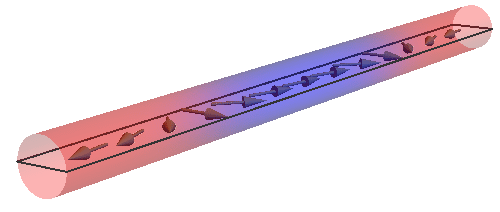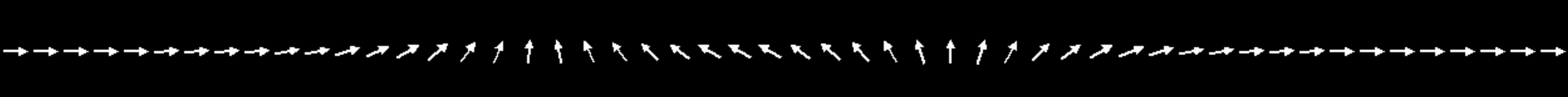# Domain walls

Back

Two domain walls in the same azimuthal plane:Two domain walls twisted by$\varphi = \frac{\pi}{2}$:Parallel Case:General Case (twisted):$\varphi = 0$$\varphi = \frac{\pi}{4}$$\varphi = \frac{\pi}{2}$$\varphi = \pi$Annihilation of parallel domain walls:Annihilation of twisted domain walls:Single domain wall (initial):Single domain wall (final):Collective coordinates:Notes:

This slideshow requires JavaScript.